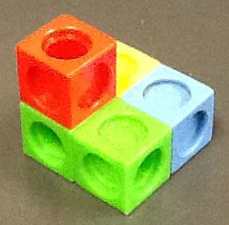# Module One Post Eight (math in drawings)

This website mostly talks about the mathematical aspects in perspective drawing. There are about three mathematical things to look for next time you see a drawing. They are the relationship between the lengths of the edges of the cubes, the angles between them and parallel and perpendicular lines.Here is the picture the website provided us. As you take a close look at the picture, you can observe the angles and lengths of the cubes. Also the way the lines point and how the angles are, looking this way at the cubes.

https://nrich.maths.org/8399